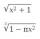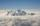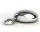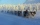# Brackets 2

Add parenthesis to make true:

5-2×6-4+2=5

Result

x = (Correct answer is: 5-2×(6-(4+2)))#### Solution:Leave us a comment of example and its solution (i.e. if it is still somewhat unclear...):

Showing 0 comments:Be the first to comment!## Next similar examples:

1. With bracketCalculate (evaluate) simple mathematical expression with a negative numbers and a bracket: 13+15*5-2*(-6)
2. Expression 1What is 7+8-(5×2)+5-4+(6×(5-3)+6)-(8+10)-7+6?
3. MountainMountain has the elevation 7450 meters and in the morning is the middle portion thereof in the clouds. How many meters of height is in the sky if bellow the clouds is 2,000 meters and above clouds are two fifths of elevation of the mountain?
4. EricaErica bought 3 1/2 yards of fabric. If she uses 2/3 of the fabric, how much will she have left?
5. BarrelsPeter has 42 barrels. One-sixth of them are filled with lemonade, one third is filled with wine and half is empty. Calculate how many barrels are with each filling.
6. MidpointsTriangle whose sides are midpoints of sides of triangle ABC has a perimeter 45. How long is perimeter of triangle ABC?
7. EmployeesOf all 360 employees, there are 11/12 women. How many men work in a company?
8. Evaluate expression 2Evaluate expression with negatives: (-3)+4+(-8)+(-6)+4+(-1)
9. Combine / add termCombine like terms 4c+c-7c
10. CupcakesIn a bowl was some cupcakes. Janka ate one third and Danka ate one quarter of cupcakes. a) How many of cookies ate together? b) How many cookies remain in a bowl? Write the results as a decimal number and in notepad also as a fraction.
11. LaboratoryMang Elio went to his laboratory at 7:00am And recorded that the temperature was 11.07°C. At lunchtime, the temperature rose by 27.9°C but dropped by 8.05°C at 5:00 PM. What was the temperature at 5:00 PM?
12. OpheliaOphelia recorded the temperature of a cold store every two hours. 1. At 6am it was -4°C and at 8am it was -1°C. By how much did the temperature rise? 2. The temperature went up by 5°C in the next two hours. .What was the temperature at 10am?
13. NoontimeIn the morning temperature was 110°F. By noontime it has gone up by 15°F. What was the noon temperature. (Give your answer in °F)
14. Melting pointThe melting point of mercury is -36°F and its boiling point is 672°F. What is the difference between the boiling point and the melting point?
15. The temperartureThe temperarture is 0 degrees. It drops 5 degrees after 1 hour and then drops 3 degrees more the next hour. What is the temperature after 6 hours?
16. 8 degrees8 degrees Celsius it cools down by 9 degrees Celsius. What is the resultant temperature?
17. 15 numberWhat number is smaller (greater) by 15 than its half?